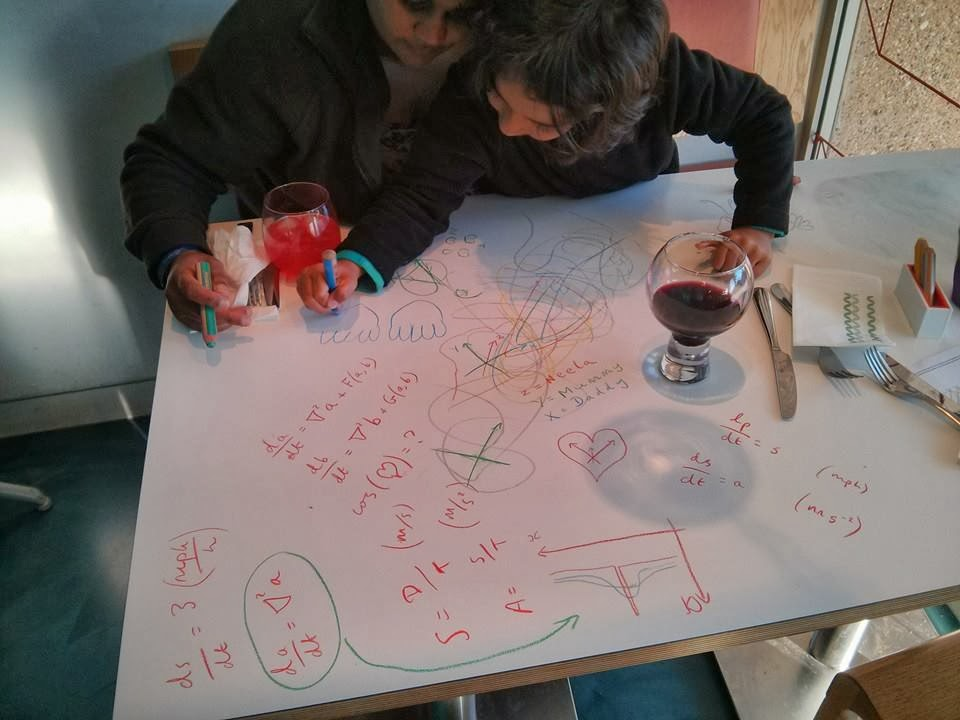An Italian restaurant where you can draw on the tables, perfect for children ...- 2013-11-04 16:03:09+0000 - Updated: 2013-11-04 16:03:09+0000
An Italian restaurant where you can draw on the tables, perfect for children of all ages. Spotting guide: XKCD reference (1 point). Heat equation (2 points). Reaction-diffusion equations (3 points). Later I showed my daughter (6) that you can add equations together: (1+3=4) + (2+3=5) = (3+6=9) and she understood it.An Italian restaurant where you can draw on the tables, perfect for children of all ages. Spotting guide: XKCD reference (1 point). Heat equation (2 points). Reaction-diffusion equations (3 points). Later I showed my daughter (6) that you can add equations together: (1+3=4) + (2+3=5) = (3+6=9) and she understood it.

Shared with: Public

This post was originally on Google+